# 一个行人行走激励下的悬索桥动力模型A Suspension Footbridge Model under Crowd-Induced Excitation

DOI: 10.12677/HJCE.2019.81010, PDF, HTML, XML, 下载: 389  浏览: 1,647  国家自然科学基金支持

Abstract: In this paper “the plane bifilar pendulum model” is proposed to study vibration of a suspension footbridge under crowd excitation. We use a plane bifilar pendulum to describe a suspension bridge by considering its structural features, which consists of two strings and a central rigid body representing the cables and deck of the footbridge, respectively. In addition, the vertical and lateral forces exerted by crowd on the deck both are considered to be harmonic with constant amplitudes. According to Lagrange method, we found that the dynamic behavior of the suspension footbridge under crowd-induced excitation can be described by a Hill equation. The solution and its stability of the plane pendulum model are theoretically analyzed based on the perturbation method, the correctness of which is verified by numerical simulations. By applying the analytical results to the London Millennium Bridge, we can easily explain the occurrence of excessive lateral vibration with 0.48 and 0.96 Hz and the “lock-in” phenomenon. Our research suggests that structural features of a suspension footbridge should not be ignored in the investigation of the pedestrian-footbridge interaction.

1. 引言

2. 平面双线摆模型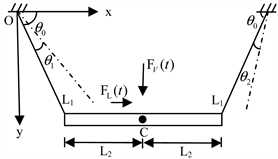Figure 1. The plane bifilar pendulum model

${\left[\text{2}{L}_{2}+\text{2}{L}_{1}\mathrm{cos}{\theta }_{0}-{L}_{1}\mathrm{cos}\left({\theta }_{0}-{\theta }_{2}\right)-{L}_{1}\mathrm{cos}\left({\theta }_{0}+{\theta }_{1}\right)\right]}^{\text{2}}+{L}_{1}{}^{2}{\left[\mathrm{sin}\left({\theta }_{0}-{\theta }_{2}\right)-\mathrm{sin}\left({\theta }_{0}+{\theta }_{1}\right)\right]}^{2}={\left(2{L}_{2}\right)}^{2}.$ (1)

$\frac{\text{d}{\theta }_{2}}{\text{d}{\theta }_{1}}=\frac{2\left({L}_{1}\mathrm{cos}{\theta }_{0}+{L}_{2}\right)\mathrm{sin}\left({\theta }_{0}+{\theta }_{1}\right)-p}{2\left({L}_{1}\mathrm{cos}{\theta }_{0}+{L}_{2}\right)\mathrm{sin}\left({\theta }_{0}-{\theta }_{2}\right)-p},$ (2)

${x}_{C}=\frac{x{}_{1}+x{}_{2}}{2},{y}_{C}=\frac{y{}_{1}+y{}_{2}}{2},$

${x}_{\text{1}}={L}_{1}\mathrm{cos}\left({\theta }_{0}+{\theta }_{1}\right),{x}_{2}=2\left({L}_{1}\mathrm{cos}{\theta }_{0}+{L}_{2}\right)-{L}_{1}\mathrm{cos}\left({\theta }_{0}-{\theta }_{2}\right),$

${y}_{1}={L}_{1}\mathrm{sin}\left({\theta }_{0}+{\theta }_{1}\right)$${y}_{\text{2}}={L}_{1}\mathrm{sin}\left({\theta }_{0}-{\theta }_{2}\right)$

$\mathrm{sin}{\theta }_{C}={L}_{1}\left(\mathrm{sin}\left({\theta }_{0}-{\theta }_{2}\right)-\mathrm{sin}\left({\theta }_{0}+{\theta }_{1}\right)\right)/\left(2{L}_{2}\right).$

$\begin{array}{l}T=\frac{1}{2}m\left({\stackrel{˙}{x}}_{C}^{2}+{\stackrel{˙}{y}}_{C}^{2}\right)+\frac{1}{2}{J}_{C}{\stackrel{˙}{\theta }}_{C}^{2}=\frac{1}{2}\left(m{P}_{1}\left({\theta }_{1},{\theta }_{2}\right)+{J}_{C}{P}_{2}\left({\theta }_{1},{\theta }_{2}\right)\right){\stackrel{˙}{\theta }}_{\text{1}}^{2},\\ V=-mg{y}_{C}=-\frac{mg{L}_{1}}{2}\left[\mathrm{sin}\left({\theta }_{0}+{\theta }_{1}\right)+\mathrm{sin}\left({\theta }_{0}-{\theta }_{2}\right)\right],\end{array}$ (3)

$\begin{array}{l}{P}_{1}\left({\theta }_{1},{\theta }_{2}\right)=\frac{{L}_{1}^{2}}{4}\left[1+{\left(\frac{\text{d}{\theta }_{2}}{\text{d}{\theta }_{1}}\right)}^{2}-2\mathrm{cos}\left(2{\theta }_{0}+{\theta }_{1}-{\theta }_{2}\right)\frac{\text{d}{\theta }_{2}}{\text{d}{\theta }_{1}}\right],\\ {P}_{2}\left({\theta }_{1},{\theta }_{2}\right)={\left[\frac{{L}_{1}\mathrm{sin}\left(2{\theta }_{0}+{\theta }_{1}-{\theta }_{2}\right)}{2\left({L}_{1}\mathrm{cos}{\theta }_{0}+{L}_{2}\right)\mathrm{sin}\left({\theta }_{0}-{\theta }_{2}\right)-{L}_{1}\mathrm{sin}\left(2{\theta }_{0}+{\theta }_{1}-{\theta }_{2}\right)}\right]}^{2}.\end{array}$

${\stackrel{¨}{\theta }}_{1}+W\left({\theta }_{1},{\stackrel{˙}{\theta }}_{1},{\theta }_{2}\right)=Q,$ (4)

$W\left({\theta }_{1},{\stackrel{˙}{\theta }}_{1},{\theta }_{2}\right)=\frac{\left[m\left(\frac{\partial {P}_{1}}{\partial {\theta }_{1}}+\frac{\partial {P}_{1}}{\partial {\theta }_{2}}\frac{\text{d}{\theta }_{2}}{\text{d}{\theta }_{1}}\right)+{J}_{C}\left(\frac{\partial {P}_{2}}{\partial {\theta }_{1}}+\frac{\partial {P}_{2}}{\partial {\theta }_{2}}\frac{\text{d}{\theta }_{2}}{\text{d}{\theta }_{1}}\right)\right]}{2\left[m{P}_{1}\left({\theta }_{1},{\theta }_{2}\right)+{J}_{C}{P}_{2}\left({\theta }_{1},{\theta }_{2}\right)\right]}{\stackrel{˙}{\theta }}_{{}_{1}}^{2}-\frac{mg{L}_{1}\left[\mathrm{cos}\left({\theta }_{0}+{\theta }_{1}\right)-\mathrm{cos}\left({\theta }_{0}-{\theta }_{2}\right)\frac{\text{d}{\theta }_{2}}{\text{d}{\theta }_{1}}\right]}{2\left[m{P}_{1}\left({\theta }_{1},{\theta }_{2}\right)+{J}_{C}{P}_{2}\left({\theta }_{1},{\theta }_{2}\right)\right]},$

Q代表广义力，由三部分构成 $Q={Q}_{V}+{Q}_{L}+{Q}_{R}$ ，其中

${Q}_{V}=\frac{{F}_{V}\left(t\right){L}_{1}\left[\mathrm{cos}\left({\theta }_{0}+{\theta }_{1}\right)-\mathrm{cos}\left({\theta }_{0}-{\theta }_{2}\right)\frac{\text{d}{\theta }_{2}}{\text{d}{\theta }_{1}}\right]}{2\left[m{P}_{1}\left({\theta }_{1},{\theta }_{2}\right)+{J}_{C}{P}_{2}\left({\theta }_{1},{\theta }_{2}\right)\right]},{Q}_{L}=-\frac{{F}_{L}\left(t\right){L}_{1}\left[\mathrm{sin}\left({\theta }_{0}+{\theta }_{1}\right)+\mathrm{sin}\left({\theta }_{0}-{\theta }_{2}\right)\frac{\text{d}{\theta }_{2}}{\text{d}{\theta }_{1}}\right]}{2\left[m{P}_{1}\left({\theta }_{1},{\theta }_{2}\right)+{J}_{C}{P}_{2}\left({\theta }_{1},{\theta }_{2}\right)\right]},$

$\begin{array}{c}{Q}_{R}=-\frac{{C}_{L}{L}_{1}^{2}{\left[\mathrm{sin}\left({\theta }_{0}+{\theta }_{1}\right)+\mathrm{sin}\left({\theta }_{0}-{\theta }_{2}\right)\frac{\text{d}{\theta }_{2}}{\text{d}{\theta }_{1}}\right]}^{2}}{4\left[m{P}_{1}\left({\theta }_{1},{\theta }_{2}\right)+{J}_{C}{P}_{2}\left({\theta }_{1},{\theta }_{2}\right)\right]}{\stackrel{˙}{\theta }}_{1}-\frac{{C}_{V}{L}_{1}^{2}{\left[\mathrm{cos}\left({\theta }_{0}+{\theta }_{1}\right)-\mathrm{cos}\left({\theta }_{0}-{\theta }_{2}\right)\frac{\text{d}{\theta }_{2}}{\text{d}{\theta }_{1}}\right]}^{2}}{4\left[m{P}_{1}\left({\theta }_{1},{\theta }_{2}\right)+{J}_{C}{P}_{2}\left({\theta }_{1},{\theta }_{2}\right)\right]}{\stackrel{˙}{\theta }}_{1}\\ -\frac{{C}_{{\theta }_{C}}{P}_{2}\left({\theta }_{1},{\theta }_{2}\right)}{m{P}_{1}\left({\theta }_{1},{\theta }_{2}\right)+{J}_{C}{P}_{2}\left({\theta }_{1},{\theta }_{2}\right)}{\stackrel{˙}{\theta }}_{1}.\end{array}$

${\theta }_{1}={\theta }_{2}=0$ 显然是系统(4)的平衡位置。考虑 ${\theta }_{1,\text{2}}$ 很小，只在0附近发生微小的变化。将(4)在平衡位置附近线性化，可得

${\stackrel{¨}{\theta }}_{1}+\text{2}{\alpha }_{\text{1}}{\stackrel{˙}{\theta }}_{1}+\left({\alpha }_{\text{2}}+{\alpha }_{\text{3}}{F}_{L}\left(t\right)+{\alpha }_{\text{4}}{F}_{V}\left(t\right)\right){\theta }_{1}={\alpha }_{\text{5}}{F}_{L}\left(t\right),$ (5)

${\alpha }_{\text{1}}=\frac{\text{1}}{\text{2}}\frac{{C}_{L}{L}_{2}^{2}{\mathrm{sin}}^{2}{\theta }_{0}+{C}_{{\theta }_{C}}{\mathrm{cos}}^{2}{\theta }_{0}}{m{L}_{2}^{2}{\mathrm{sin}}^{2}{\theta }_{0}+{J}_{C}{\mathrm{cos}}^{2}{\theta }_{0}},{\alpha }_{\text{2}}=\frac{mg\left({L}_{2}+{L}_{1}{\mathrm{cos}}^{3}{\theta }_{0}\right){\left({L}_{2}+{L}_{1}-{L}_{1}\mathrm{cos}{\theta }_{0}\right)}^{2}}{m{L}_{1}{L}_{2}{\mathrm{sin}}^{3}{\theta }_{0}{\left({L}_{2}+{L}_{1}-{L}_{1}\mathrm{cos}{\theta }_{0}\right)}^{2}+{J}_{C}{L}_{1}{L}_{2}\mathrm{sin}{\theta }_{0}{\mathrm{cos}}^{2}{\theta }_{0}},$

${\alpha }_{\text{3}}=\frac{{L}_{\text{2}}\mathrm{cos}{\theta }_{0}\left({L}_{\text{2}}+{L}_{1}\mathrm{cos}{\theta }_{0}\right)}{{L}_{1}\left(m{L}_{2}^{2}{\mathrm{sin}}^{2}{\theta }_{0}+{J}_{C}{\mathrm{cos}}^{2}{\theta }_{0}\right)},{\alpha }_{\text{4}}=\frac{{L}_{\text{2}}\left({L}_{\text{2}}+{L}_{1}{\mathrm{cos}}^{3}{\theta }_{0}\right)}{{L}_{1}\mathrm{sin}{\theta }_{0}\left(m{L}_{2}^{2}{\mathrm{sin}}^{2}{\theta }_{0}+{J}_{C}{\mathrm{cos}}^{2}{\theta }_{0}\right)},{\alpha }_{\text{5}}=-\frac{{L}_{2}^{2}\mathrm{sin}{\theta }_{0}}{{L}_{1}\left(m{L}_{2}^{2}{\mathrm{sin}}^{2}{\theta }_{0}+{J}_{C}{\mathrm{cos}}^{2}{\theta }_{0}\right)}.$

${F}_{i}\left(t\right)=\lambda {\alpha }_{i}g{m}_{p}\left(x\right)\mathrm{cos}\left({\Omega }_{i}t\right),i=V,L,$

${\stackrel{¨}{\theta }}_{1}+2\xi {\stackrel{˙}{\theta }}_{1}+\left(\upsilon -\epsilon \mathrm{cos}\tau -\eta \mathrm{cos}\left(2\tau \right)\right){\theta }_{1}=\kappa \epsilon \mathrm{cos}\tau ,$ (6)

$\xi =\frac{{\alpha }_{1}}{{\Omega }_{L}},\upsilon =\frac{{\alpha }_{2}}{{\Omega }_{L}^{2}}=\frac{{\omega }^{2}}{{\Omega }_{L}^{2}},\epsilon =\frac{{\alpha }_{3}\lambda {\alpha }_{L}g{m}_{p}\left(x\right)}{{\Omega }_{L}^{2}},\eta =\frac{{\alpha }_{4}\lambda {\alpha }_{V}g{m}_{p}\left(x\right)}{{\Omega }_{L}^{2}},\mu =\frac{{\Omega }_{V}}{{\Omega }_{L}},\kappa =-\frac{{L}_{2}{\mathrm{sin}}^{2}{\theta }_{0}}{{L}_{2}+{L}_{1}{\mathrm{cos}}^{3}{\theta }_{0}}.$

$\stackrel{¨}{y}+\left(\delta -\psi \left(\tau \right)\right)y=\kappa \epsilon \mathrm{cos}\tau ,$ (7)

(7)是2次简谐模式的Hill方程。由(5)和(6)可知，参数 $\epsilon$$\eta$ 都是小参数。下节中将讨论(7)的通解。

3. 平面双线摆控制方程的通解

$y={y}_{0}+\epsilon {y}_{11}+\eta {y}_{12}+{\epsilon }^{2}{y}_{21}+{\eta }^{2}{y}_{22}+\epsilon \eta {y}_{23}+\cdot \cdot \cdot .$ (8)

$\begin{array}{l}{\stackrel{¨}{y}}_{0}+\delta {y}_{0}=0,\\ {\stackrel{¨}{y}}_{11}+\delta {y}_{11}=\left(\kappa -{y}_{0}\right)\mathrm{cos}\tau ，\\ {\stackrel{¨}{y}}_{12}+{\delta }_{0}{y}_{12}=-{y}_{0}\mathrm{cos}\left(2\tau \right).\end{array}$ (9)

(9)中第一式的通解可以写为

${y}_{0}=a\mathrm{cos}\left(\sqrt{\delta }t\right)+b\mathrm{sin}\left(\delta t\right)$(10)

$\begin{array}{c}{y}_{12}=\frac{1}{8\left(\delta -1\right)}\\ \left(-\left(\sqrt{\delta }+1\right)\left(a\mathrm{cos}\left(\sqrt{\delta }\tau -2\tau \right)+b\mathrm{sin}\left(\sqrt{\delta }\tau -2\tau \right)\right)+\left(\sqrt{\delta }-1\right)\left(a\mathrm{cos}\left(\sqrt{\delta }\tau +2\tau \right)+b\mathrm{sin}\left(\sqrt{\delta }\tau +2\tau \right)\right)\right).\end{array}$ (11)

4. 平面双线摆系统的稳定性分析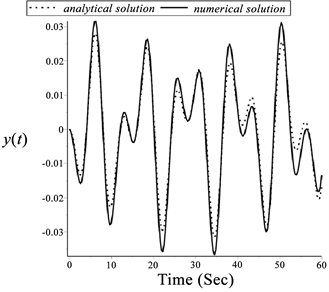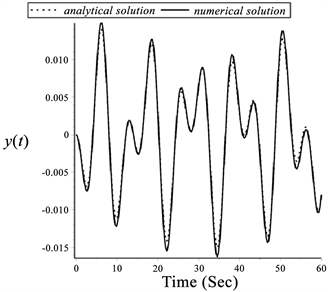(a) $\delta =\text{0}\text{.3}$ , $\epsilon =0.01$ , $\eta =\text{0}\text{.1}$ , $\kappa =-\text{0}\text{.5}$ (b) $\delta =\text{0}\text{.3}$ , $\epsilon =0.\text{02}$ , $\eta =\text{0}\text{.2}$ , $\kappa =-\text{0}\text{.5}$

Figure 2. Comparison between the first order analytical solution (···dot line) and the numerical solution (— solid line)

$\delta ={\delta }_{\text{0}}+\epsilon {\delta }_{\text{11}}+\eta {\delta }_{\text{12}}+{\epsilon }^{\text{2}}{\delta }_{\text{21}}+{\eta }^{\text{2}}{\delta }_{\text{22}}+\epsilon \eta {\delta }_{\text{23}}+\cdot \cdot \cdot ,$

$\begin{array}{l}{\stackrel{¨}{y}}_{0}+{\delta }_{0}{y}_{0}=0,\\ {\stackrel{¨}{y}}_{11}+{\delta }_{0}{y}_{11}=\kappa \mathrm{cos}\tau -\left({\delta }_{11}+\mathrm{cos}\tau \right){y}_{0}，\\ {\stackrel{¨}{y}}_{12}+{\delta }_{0}{y}_{12}=-\left({\delta }_{12}+\mathrm{cos}\left(2\tau \right)\right){y}_{0},\\ {\stackrel{¨}{y}}_{21}+{\delta }_{0}{y}_{21}=-{\delta }_{21}{y}_{0}-\left({\delta }_{11}+\mathrm{cos}\left(\tau \right)\right){y}_{11},\\ {\stackrel{¨}{y}}_{22}+{\delta }_{0}{y}_{22}=-{\delta }_{22}{y}_{0}-\left({\delta }_{12}+\mathrm{cos}\left(2\tau \right)\right){y}_{12},\end{array}$ (12)

${\stackrel{¨}{y}}_{23}+{\delta }_{0}{y}_{23}=-{\delta }_{23}{y}_{0}-\left({\delta }_{12}+\mathrm{cos}\left(2\tau \right)\right){y}_{11}-\left({\delta }_{11}+\mathrm{cos}\tau \right){y}_{12},$

4.1. δ接近1/4

$\delta \text{=}1/4$ 带入(12)的第一式，可以解得

${y}_{0}=a\mathrm{cos}\frac{\tau }{2}+b\mathrm{sin}\frac{\tau }{2},$ (13)

${\delta }_{\text{11}}=\frac{\text{1}}{\text{2}}\text{\hspace{0.17em}}\text{and}\text{\hspace{0.17em}}b=0$ 或者 ${\delta }_{11}=-\frac{1}{2}\text{\hspace{0.17em}}\text{and}\text{\hspace{0.17em}}a=0$

4.1.1. ${\delta }_{11}=\frac{1}{2}\text{\hspace{0.17em}}and\text{\hspace{0.17em}}b=0$

${y}_{11}=-\frac{1}{4}a\mathrm{cos}\frac{3\tau }{2}+\frac{4}{3}\kappa \mathrm{cos}\tau .$

${y}_{12}=-\frac{1}{12}a\left(3\mathrm{cos}\frac{3\tau }{2}+\mathrm{cos}\frac{5\tau }{2}\right).$

$\begin{array}{l}{\delta }_{\text{12}}=-\frac{1}{8},\\ {y}_{21}=\frac{1}{48}a\left(-3\mathrm{cos}\frac{3\tau }{2}+\mathrm{cos}\frac{5\tau }{2}\right)+\frac{8}{45}\kappa \left(15+\text{5}\mathrm{cos}\tau -\mathrm{cos}\left(2\tau \right)\right),\\ {\delta }_{22}=-\frac{1}{6},\\ {y}_{22}=\frac{1}{480}a\left(5\mathrm{cos}\frac{7\tau }{2}+\mathrm{cos}\frac{9\tau }{2}\right),\\ {\delta }_{23}=-\frac{1}{4},\end{array}$

${y}_{23}=\frac{1}{72}a\left(-3\mathrm{cos}\frac{3\tau }{2}+\mathrm{cos}\frac{5\tau }{2}+\mathrm{cos}\frac{7\tau }{2}\right)-\frac{8}{3}\kappa \left(\frac{1}{3}\mathrm{cos}\tau +\frac{1}{35}\mathrm{cos}\left(3\tau \right)\right).$

$\delta =\frac{1}{4}+\frac{1}{2}\epsilon -\frac{1}{8}{\epsilon }^{2}-\frac{1}{6}{\eta }^{2}-\frac{1}{4}\epsilon \eta .$ (14)

4.1.2. ${\delta }_{11}=-\frac{1}{2}\text{\hspace{0.17em}}and\text{\hspace{0.17em}}a=0$

$\delta =\frac{1}{4}-\frac{1}{2}\epsilon -\frac{1}{8}{\epsilon }^{2}-\frac{1}{6}{\eta }^{2}+\frac{1}{4}\epsilon \eta .$ (15)

$\begin{array}{c}y=b\mathrm{sin}\frac{\tau }{2}-\left(\frac{1}{4}b\mathrm{sin}\frac{3\tau }{2}-\frac{4}{3}\kappa \mathrm{cos}\tau \right)\epsilon -\frac{1}{12}b\left(-3\mathrm{sin}\frac{3\tau }{2}+\mathrm{sin}\frac{5\tau }{2}\right)\eta \\ +\left[\frac{1}{48}b\left(3\mathrm{sin}\frac{3\tau }{2}+\mathrm{sin}\frac{5\tau }{2}\right)-\frac{8}{45}\kappa \left(-15+5\mathrm{cos}\tau +\mathrm{cos}\left(2\tau \right)\right)\right]{\epsilon }^{2}+\frac{1}{480}b\left(-5\mathrm{sin}\frac{7\tau }{2}+\mathrm{sin}\frac{9\tau }{2}\right){\eta }^{2}\\ +\left[\frac{1}{72}b\left(-3\mathrm{sin}\frac{3\tau }{2}-\mathrm{sin}\frac{5\tau }{2}+\mathrm{sin}\frac{7\tau }{2}\right)-\frac{8}{3}\kappa \left(\frac{1}{3}\mathrm{cos}\tau +\frac{1}{35}\mathrm{cos}\left(3\tau \right)\right)\right]\epsilon \eta .\end{array}$ (16)

4.2. δ接近1

$\delta =\text{1}-\frac{\kappa }{a}\epsilon +\frac{1}{2}\eta +\frac{5}{12}{\epsilon }^{2}-\frac{1}{32}{\eta }^{2}.$ (17)

$\begin{array}{c}y=a\mathrm{cos}\tau +\frac{1}{6}a\left(3-\mathrm{cos}\left(2\tau \right)\right)\epsilon -\frac{1}{16}a\mathrm{cos}\left(3\tau \right)\eta \\ +\left[\frac{1}{96}a\mathrm{cos}\left(3\tau \right)+\frac{1}{18}\kappa \left(9+\mathrm{cos}\left(2\tau \right)\right)\right]{\epsilon }^{2}+\frac{1}{768}a\left(-3\mathrm{cos}\left(3\tau \right)+\mathrm{cos}\left(5\tau \right)\right){\eta }^{2}\\ +\left[\frac{1}{1440}a\left(-265\mathrm{cos}\left(2\tau \right)+11\mathrm{cos}\left(4\tau \right)-480\right)+\frac{1}{128}\kappa \left(3\mathrm{cos}\tau +\mathrm{cos}\left(3\tau \right)\right)\right]\epsilon \eta .\end{array}$ (18)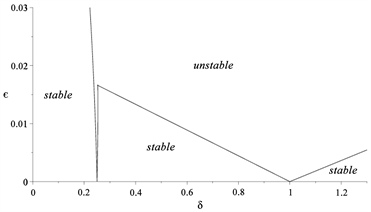(a) $a=±\text{0}\text{.001}$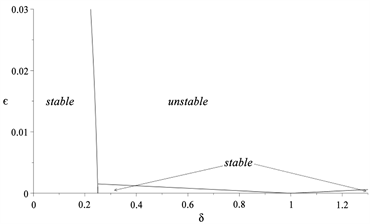(b) $a=±0.01$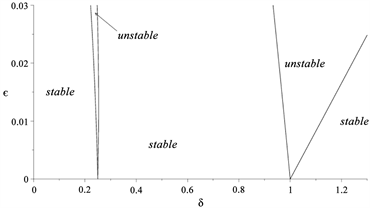(c) $a=±\text{0}\text{.02}$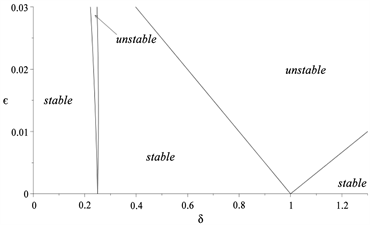(d) $a=±0.0\text{7}$

Figure 3. Transition curves of Eq. (7) in the $\delta -\epsilon$ parameter plane for $\kappa =-0.5$

$a$ 表示桥做侧向周期振动时的振幅。当 $a$ 非常小时，此时 $\epsilon$ 非常小。如图3(a)所示，此时参数平面上稳定性区域面积很小。这表明此时增加桥上人数很容易加大桥的侧向不稳定性，这会使得 $a$ 增加。随着 $a$ 增大参数平面上不稳定区域开始缩小，并逐渐收缩到 $\delta \text{=}1/4,1$ 附近(见图3(b)~(d))。根据参数  物理含义，当人数增加的足够多时不稳定情况仅仅在桥的侧向自振频率接近1/2人的侧向步频，或者接近人的竖向步频时出现。利用图3中的稳定性图片可以解释悬索桥在行人行走作用下出现的锁频现象。当桥上行人数量从零开始增加时，不管桥的侧向频率和行人激励力频率之间的关系，桥的侧向振动会迅速增加。随着桥的侧向振动幅度的增加，桥上行人会不自觉地和桥同步使得行走更为舒适。而这种同步将使得行人的竖向行走频率接近桥的侧向自振频率或者自振频率的倍数。但这恰恰是悬索桥系统稳定性被破坏的条件，因此这将最终造成悬索桥过大的侧向振动。由于行人在行走过程中不自觉地和侧向摆动的桥同步，所以这一过程几乎是无法避免的。著名的伦敦千禧桥中跨的第一阶侧向自振频率为0.48 Hz，根据上面的分析过大侧向振动会出现在行人侧向步频为1倍和2倍桥侧向自振频率附近。根据实测行人侧向力步频在0.7~1.2 Hz左右，因此千禧桥会在0.35~0.6 Hz和0.7~1.2 Hz附近出现过大的侧向振动。实际上发生侧向振动频率恰好为0.48和0.96 Hz。

5. 结论

  Bachmann, H. and Ammann, W. (1987) Structural Engineering Documents 3e, Vibrations in Structures: Induced by Man and Machines. International Association for Bridge and Structural Engineering (IABSE), Zurich, Switzer-land.  Fujino, Y., Pacheco, B.M., Nakamura, S. and Warnitchai, P. (1993) Synchornization of Human Walking Observed during Lateral Vibration of a Congested Pedestrian Bridge. Earthquake Engineering Structural Dynamics, 22, 741-758. https://doi.org/10.1002/eqe.4290220902  Dallard, P., Fitzpatrick, A.J., Flint, A., Le Bourva, S., Low, A., Ridsdill Smith, R.M. and Willford, M. (2001) The London Millennium Footbridge. Structural Engineer, 79, 17-33.  Dallard, P., Fitzpatrick, A.J., Flint, A., Low, A., Ridsdill Smith, R.M., Willford, M. and Roche, M. (2001) London Millennium Bridge: Pedestrian-Induced Lateral Vibration. Journal of Bridge Engineering, 6, 412-417. https://doi.org/10.1061/(ASCE)1084-0702(2001)6:6(412)  Roberts, T.M. (2005) Lateral Pedestrian Excitation of Footbridges. Journal of Bridge Engineering, 10, 107-112. https://doi.org/10.1061/(ASCE)1084-0702(2005)10:1(107)  Newland, D.E. (2004) Pedestrian Excitation of Bridges. Proceedings of the Institution of Mechanical Engineers, Part C: Journal of Mechanical Engineering Science, 218, 477-492. https://doi.org/10.1243/095440604323052274  Piccardo, G. and Tubino, F. (2008) Parametric Resonance of Flexible Footbridges under Crowd-Induced Lateral Excitation. Journal of Sound and Vibration, 311, 353-371. https://doi.org/10.1016/j.jsv.2007.09.008  MCRobie, A., Morgenthal, G., Lasenby, J. and Ringer, M. (2003) Section Model Tests on Human Structure Lock In. Proceedings of the Institution of Civil Engineering-Bridge Engineering, 156, 71-79. https://doi.org/10.1680/bren.2003.156.2.71  Zhou, D. and Ji, T.J. (2008) Dynamic Characteristics of a Gener-alized Suspension System. International Journal of Mechanical Sciences, 50, 30-42. https://doi.org/10.1016/j.ijmecsci.2007.05.007  Grundamann, H., Kreuzinger, H. and Schneider M. (1993) Dynamic Calculation of Footbridges. Bauingenieur-Germany, 68, 215-225.